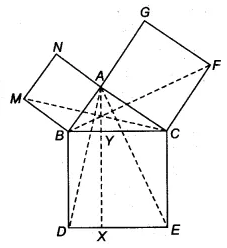Q

# In Fig. 9.34, ABC is a right triangle right angled at A. BCED, ACFG and ABMN are squares on the sides BC, CA and AB respectively. Line segment AX ⊥ DE meets BC at Y. Show that: (v)

Q : 8    In Fig. , ABC is a right triangle right angled at A. BCED, ACFG and ABMN are squares on the sides BC, CA and AB respectively. Line segment   meets BC at Y. Show that:

(v)ViewsSince ||gm CYXE and ACE lie on the same base CE and between the same parallels CE and AX.
Therefore, 2. ar(ACE) = ar (||gm CYXE)
B
ut, FCB    ACE (in iv part)
Since the congruent triangle has equal areas. So,

Hence proved

Exams
Articles
Questions